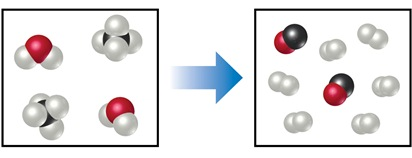# Problem: The diagram represents a high-temperature reaction between CH4 and H2O.Based on this reaction, how many moles of H2 can be obtained starting with 6 mol CH4?

###### FREE Expert Solution

We’re being asked to calculate the moles of H2 produced by the reaction of 6 mol CH4

For this problem, we need to do the following steps:

Step 1: Write and balance a chemical equation for the given reaction.

Step 2: Calculate the moles of H2 produced.

81% (153 ratings)###### Problem Details

The diagram represents a high-temperature reaction between CH4 and H2O.

Based on this reaction, how many moles of H2 can be obtained starting with 6 mol CH4?Frequently Asked Questions

What scientific concept do you need to know in order to solve this problem?

Our tutors have indicated that to solve this problem you will need to apply the Stoichiometry concept. You can view video lessons to learn Stoichiometry. Or if you need more Stoichiometry practice, you can also practice Stoichiometry practice problems.

What professor is this problem relevant for?

Based on our data, we think this problem is relevant for Professor Marks' class at ASU.